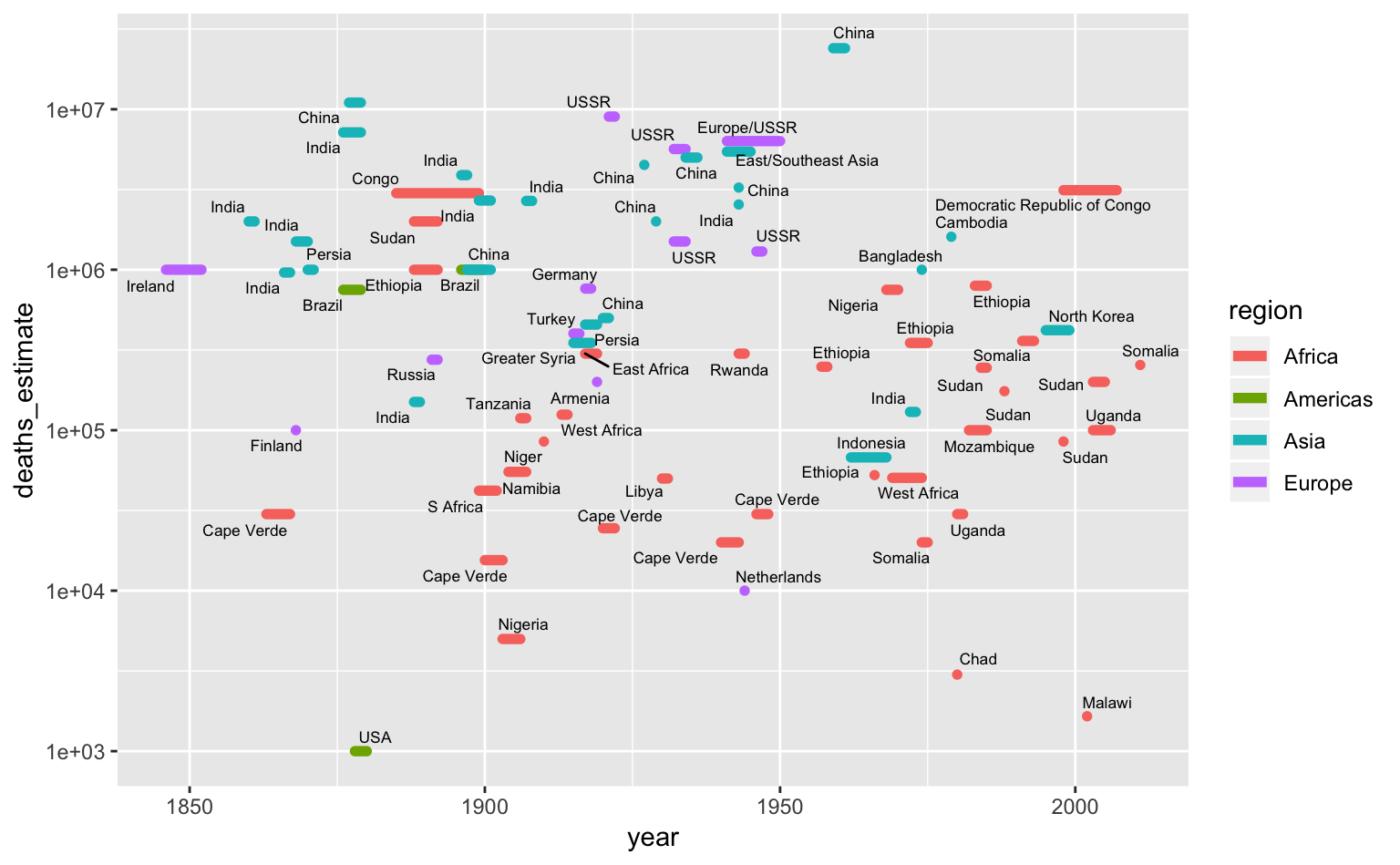# 6 Time series

``````# Libraries
library(tidyverse)
library(dcldata)``````

In the last chapter, we visualized the relationship between per capita GDP and life expectancy. You might have wondered how time fits into that association. In this chapter, we’ll explore life expectancy and GDP over time.

The following ggplot2 cheat sheet sections will be helpful for this chapter:

• Geoms
• `geom_path()`
• Scales

The lubridate package is a helpful tool for working with dates. We’ll use some lubridate functions throughout the chapter. Take a look at the lubridate cheat sheet if you’re not already familiar with the package.

Not all time series are alike. In some situations, you’ll be interested in a long-term trend, but in others you’ll want to highlight short-term changes or even just individual values. In this chapter, we’ll cover various strategies for dealing with these different scenarios.

First, we’ll talk about the mechanics of date scales, which are useful for time series.

## 6.1 Mechanics

### 6.1.1 Date/time scales

Sometimes, your time series data will include detailed date or time information stored as a date, time, or date-time. For example, the `nycflights13::flights` variable `time_hour` is a date-time.

``````nycflights13::flights %>%
select(time_hour)
#> # A tibble: 336,776 x 1
#>   time_hour
#>   <dttm>
#> 1 2013-01-01 05:00:00
#> 2 2013-01-01 05:00:00
#> 3 2013-01-01 05:00:00
#> 4 2013-01-01 05:00:00
#> 5 2013-01-01 06:00:00
#> 6 2013-01-01 05:00:00
#> # … with 3.368e+05 more rows``````

When you map `time_hour` to an aesthetic, ggplot2 uses `scale_*_datetime()`, the scale function for date-times. There is also `scale_*_date()` for dates and `scale_*_time()` for times. The date- and time-specific scale functions are useful because they create meaningful breaks and labels.

`flights_0101_0102` contains data on the number of flights per hour on January 1st and January 2nd, 2013.

``````flights_0101_0102 <-
nycflights13::flights %>%
filter(month == 1, day <= 2) %>%
group_by(time_hour = lubridate::floor_date(time_hour, "hour")) %>%
summarize(num_flights = n())

flights_0101_0102
#> # A tibble: 38 x 2
#>   time_hour           num_flights
#>   <dttm>                    <int>
#> 1 2013-01-01 05:00:00           6
#> 2 2013-01-01 06:00:00          52
#> 3 2013-01-01 07:00:00          49
#> 4 2013-01-01 08:00:00          58
#> 5 2013-01-01 09:00:00          56
#> 6 2013-01-01 10:00:00          39
#> # … with 32 more rows``````
``````flights_0101_0102 %>%
ggplot(aes(time_hour, num_flights)) +
geom_col()``````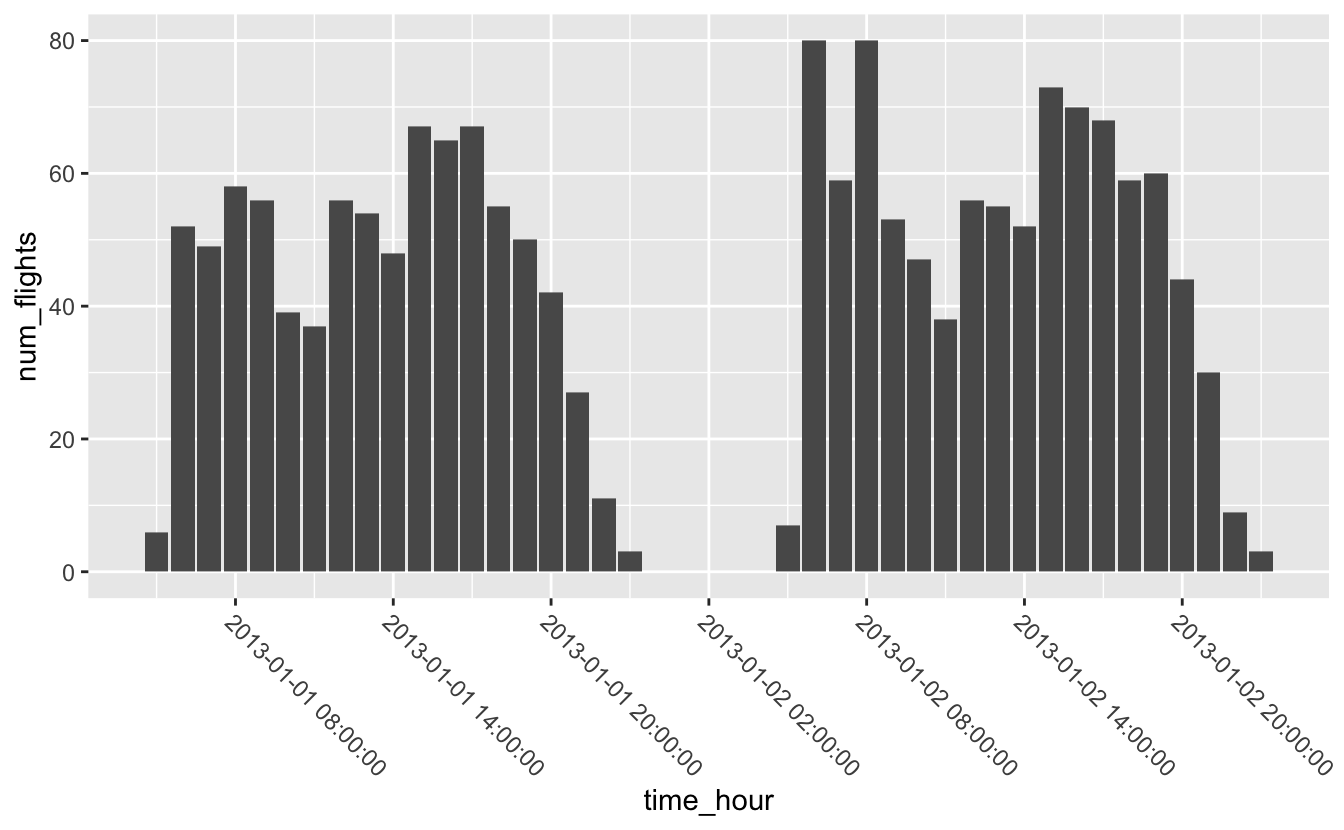Just like with the other scale functions, you can change the breaks using the `breaks` argument. `scale_*_date()` and `scale_*_datetime()` also include a `date_breaks` argument that allows you to supply the breaks in date-time units, like “1 month”, “6 years”, or “2 hours.”

``````flights_0101_0102 %>%
ggplot(aes(time_hour, num_flights)) +
geom_col() +
scale_x_datetime(date_breaks = "6 hours") +
theme(axis.text.x = element_text(angle = -45, hjust = 0))``````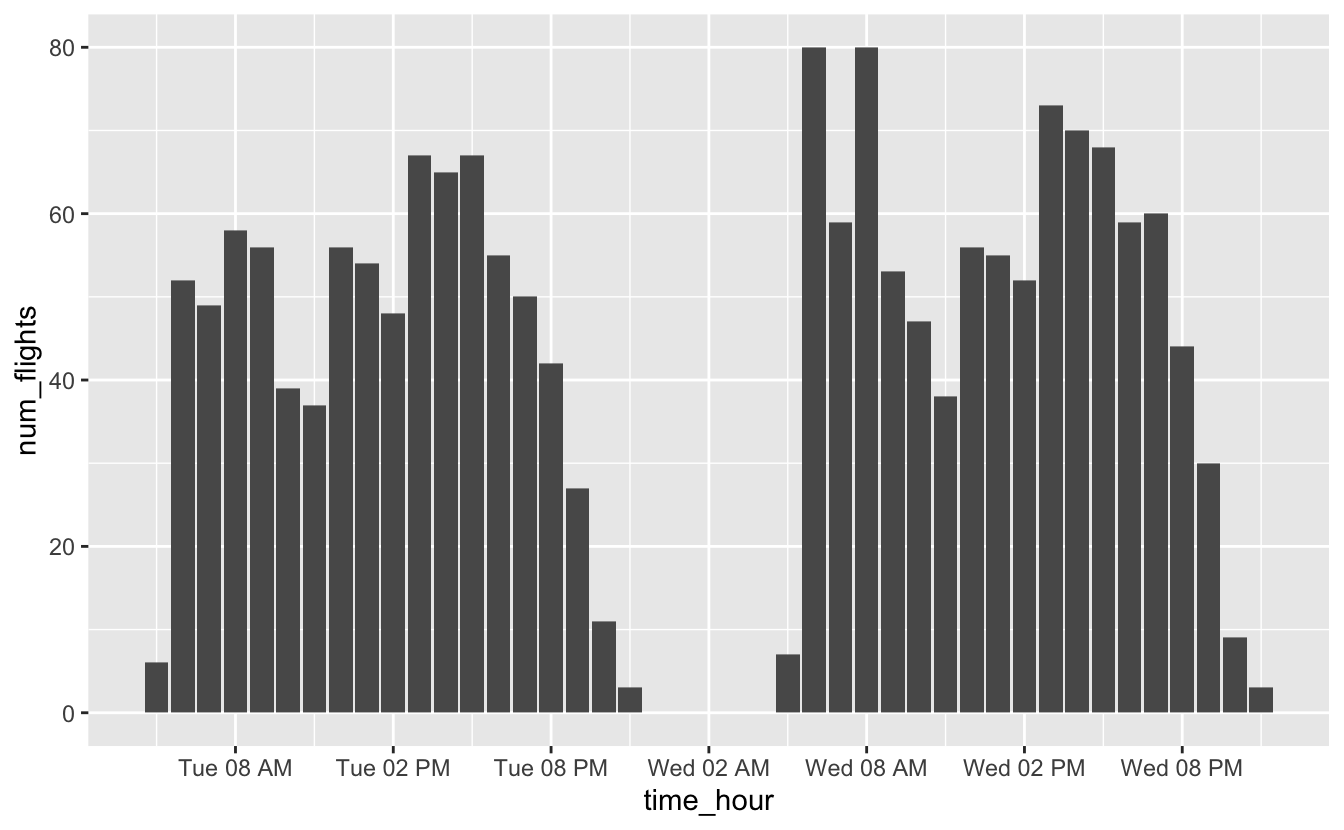Similarly, you can change the labels using the `labels` argument, but `scale_*_date()` and `scale_*_datetime()` also include a `date_labels` function made for working with dates. `date_labels` takes the same formatting strings as functions like `ymd()` and `as_datetime()`. You can see a list of all formatting strings at `?strptime`.

We’ll use `date_labels` to format `time_hour` so that it doesn’t take up as much space.

``````flights_0101_0102 %>%
ggplot(aes(time_hour, num_flights)) +
geom_col() +
scale_x_datetime(date_breaks = "6 hours", date_labels = "%a %I %p") ``````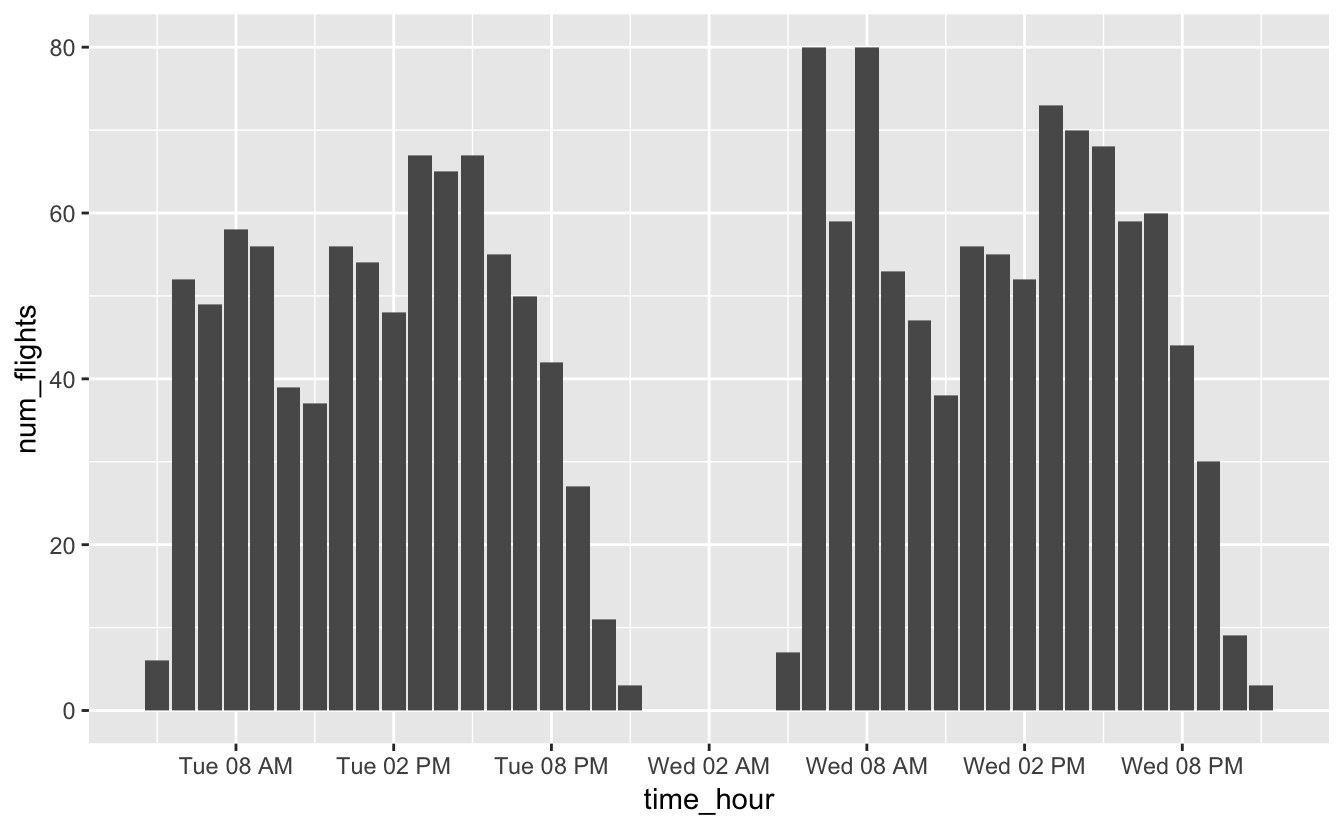## 6.3 Short-term fluctuations

In the mechanics section of this chapter, you saw the following plot.

``````flights_0101_0102 %>%
ggplot(aes(time_hour, num_flights)) +
geom_col() +
scale_x_datetime(date_breaks = "6 hours", date_labels = "%a %I %p") ``````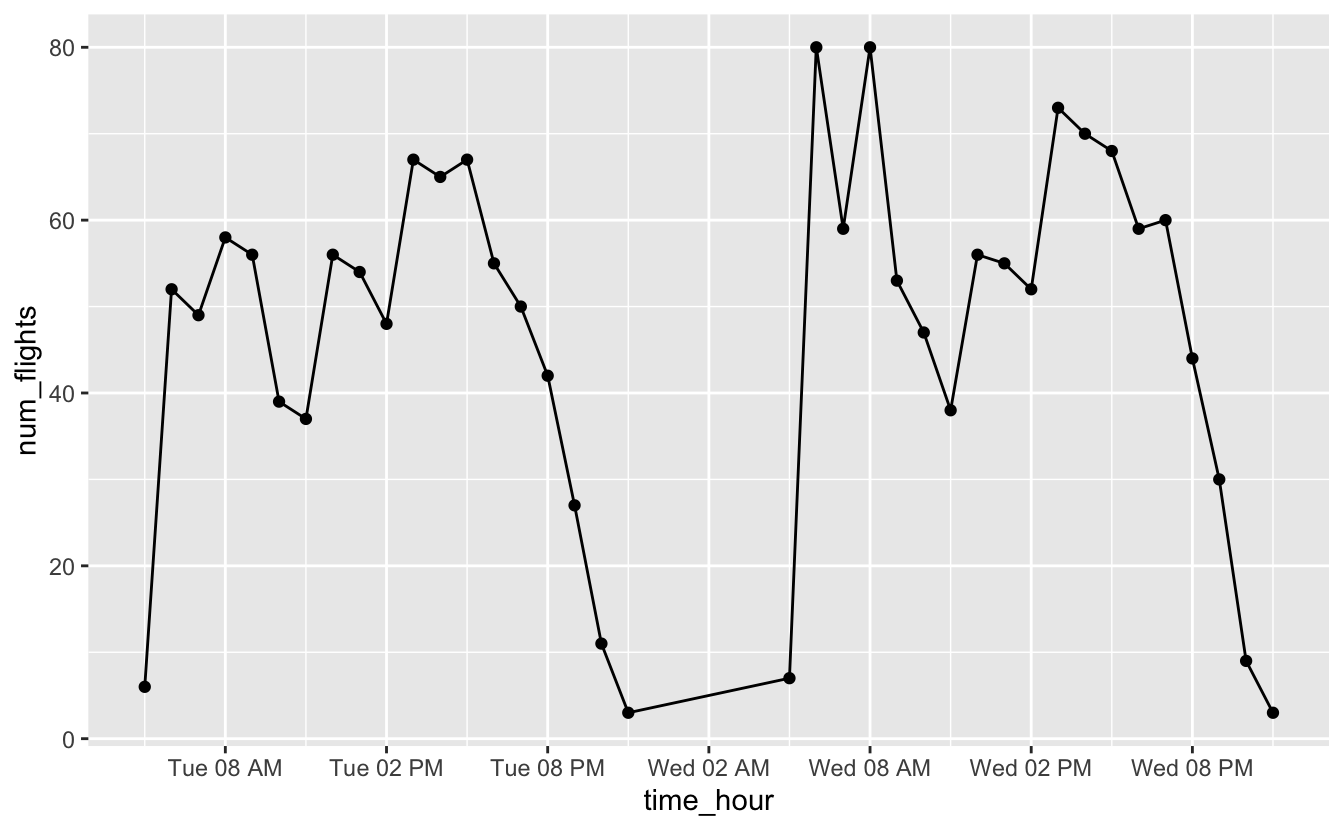You might wonder why we used `geom_col()` to represent a time series. Here’s the same plot using `geom_line()` and `geom_point()`.

``````flights_0101_0102 %>%
ggplot(aes(time_hour, num_flights)) +
geom_line() +
geom_point() +
scale_x_datetime(date_breaks = "6 hours", date_labels = "%a %I %p")``````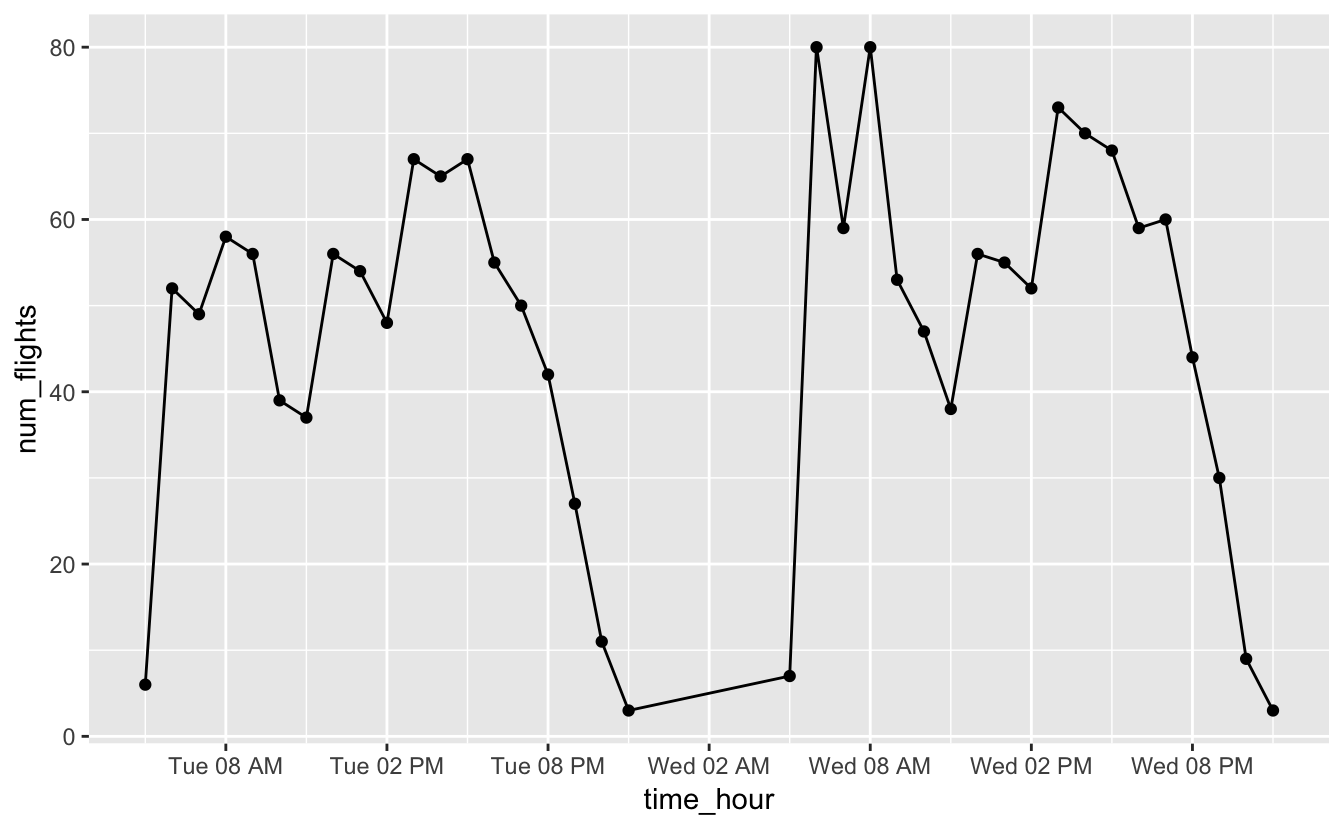From both plots, you can see that most flights occur in the early morning and around 4pm, but notice that we’re actually treating time like a discrete variable in this situation. We’ve counted the number of flights for each hour, and so it’s useful to be able to connect a number of flights with a specific hour. Columns make it easier to connect numbers of flights to specific hours.

Vertical segment plots using `geom_segment()` can also be helpful for some time series data. Say we want to understand what the first week in January looked like.

``````flights_week_1 <-
nycflights13::flights %>%
filter(lubridate::week(time_hour) == 1) %>%
group_by(time_hour = lubridate::floor_date(time_hour, "hour")) %>%
summarize(num_flights = n())``````

`geom_point()` and `geom_line()` produce the following plot.

``````flights_week_1 %>%
ggplot(aes(time_hour, y = num_flights)) +
geom_line() +
geom_point() +
scale_x_datetime(date_breaks = "1 day", date_labels = "%a") ``````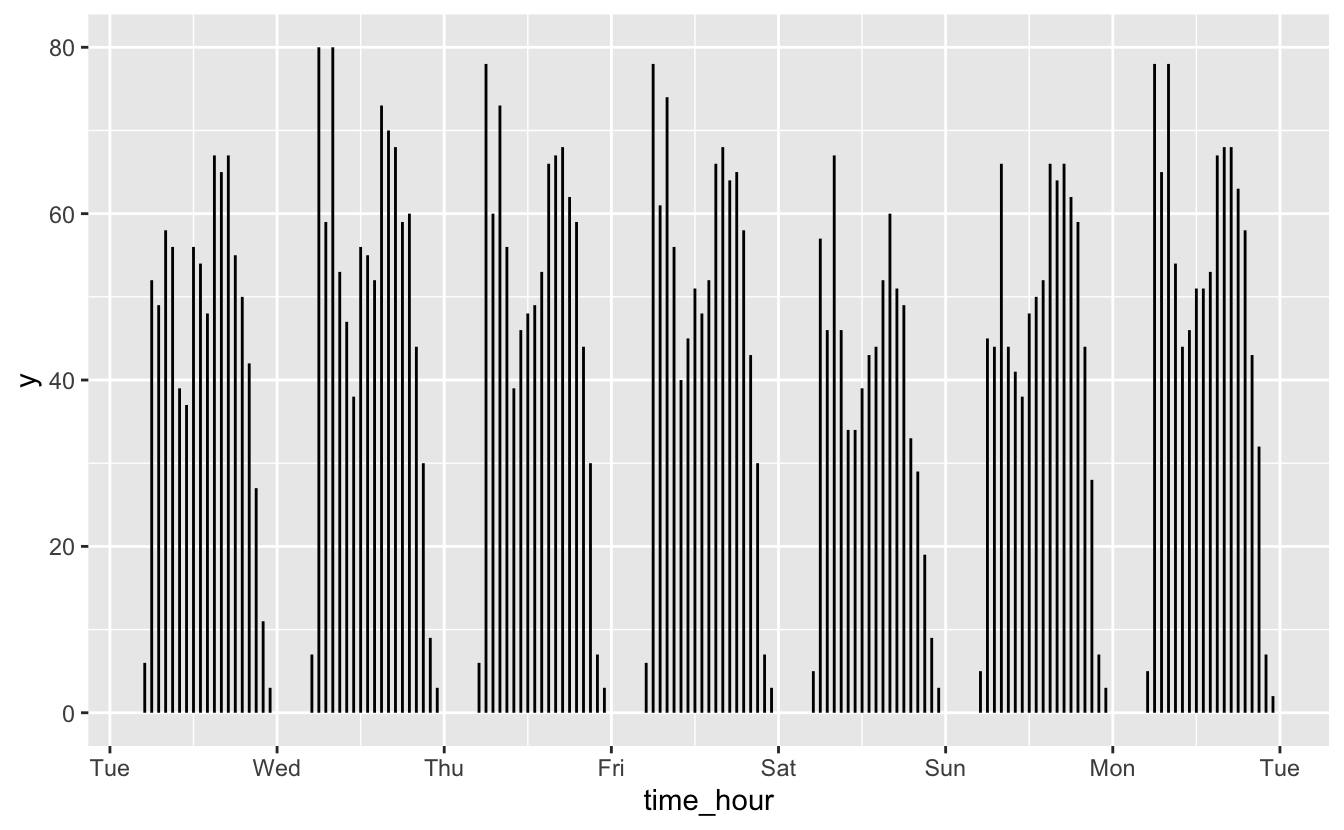You can see that each day is shaped similarly. However, you can’t tell that there are actually no flights for a couple hours each night.

``````flights_week_1 %>%
ggplot() +
geom_segment(
aes(x = time_hour, xend = time_hour, y = num_flights, yend = 0)
) +
scale_x_datetime(date_breaks = "1 day", date_labels = "%a") ``````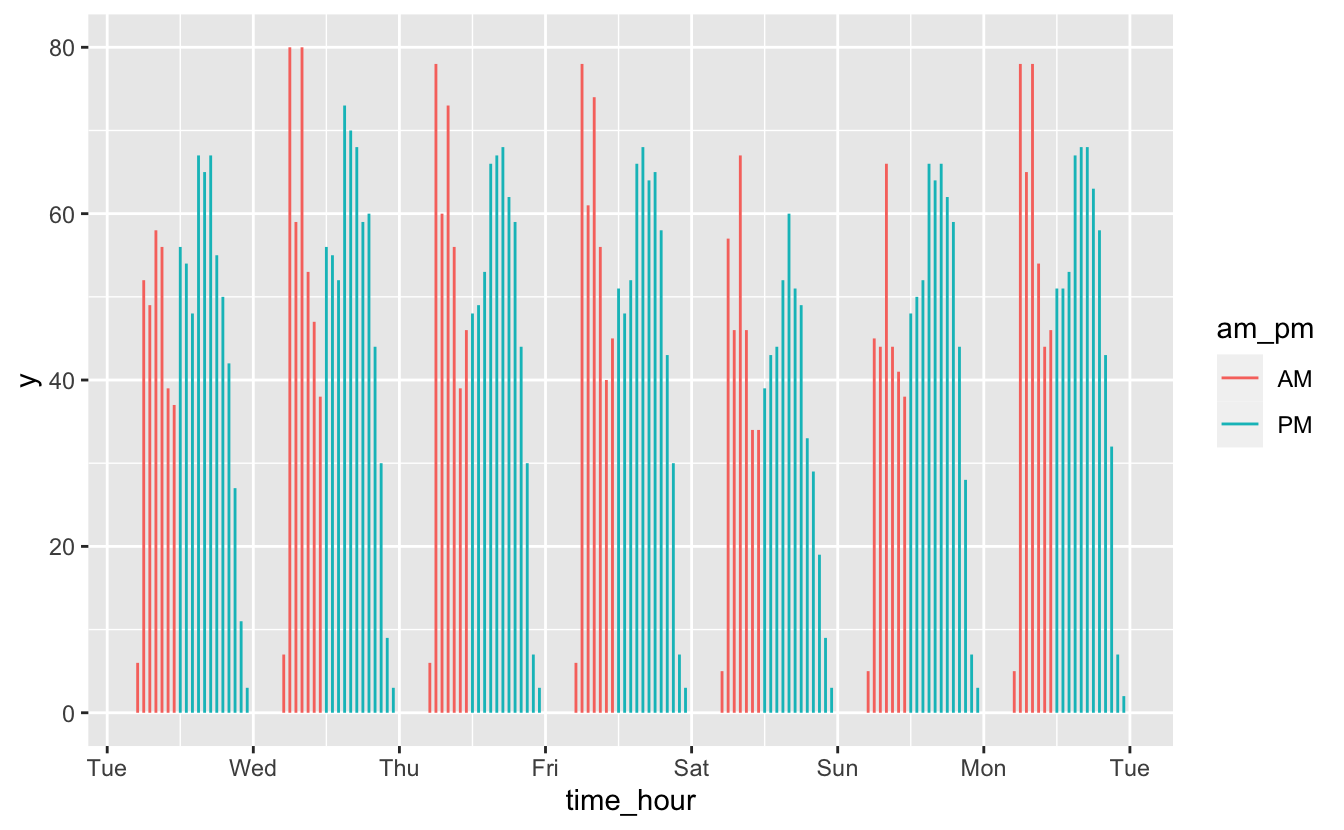`geom_segment()` does a better job of showing the gaps between days. Segments also make it easier to perceive each day as a group to compare against the others. Another advantage of `geom_segment()` is that we can use `color` to encode a categorical variable.

``````flights_week_1 %>%
mutate(am_pm = if_else(lubridate::am(time_hour), "AM", "PM")) %>%
ggplot() +
geom_segment(
aes(
x = time_hour,
xend = time_hour,
y = num_flights,
yend = 0,
color = am_pm
)
) +
scale_x_datetime(date_breaks = "1 day", date_labels = "%a") ``````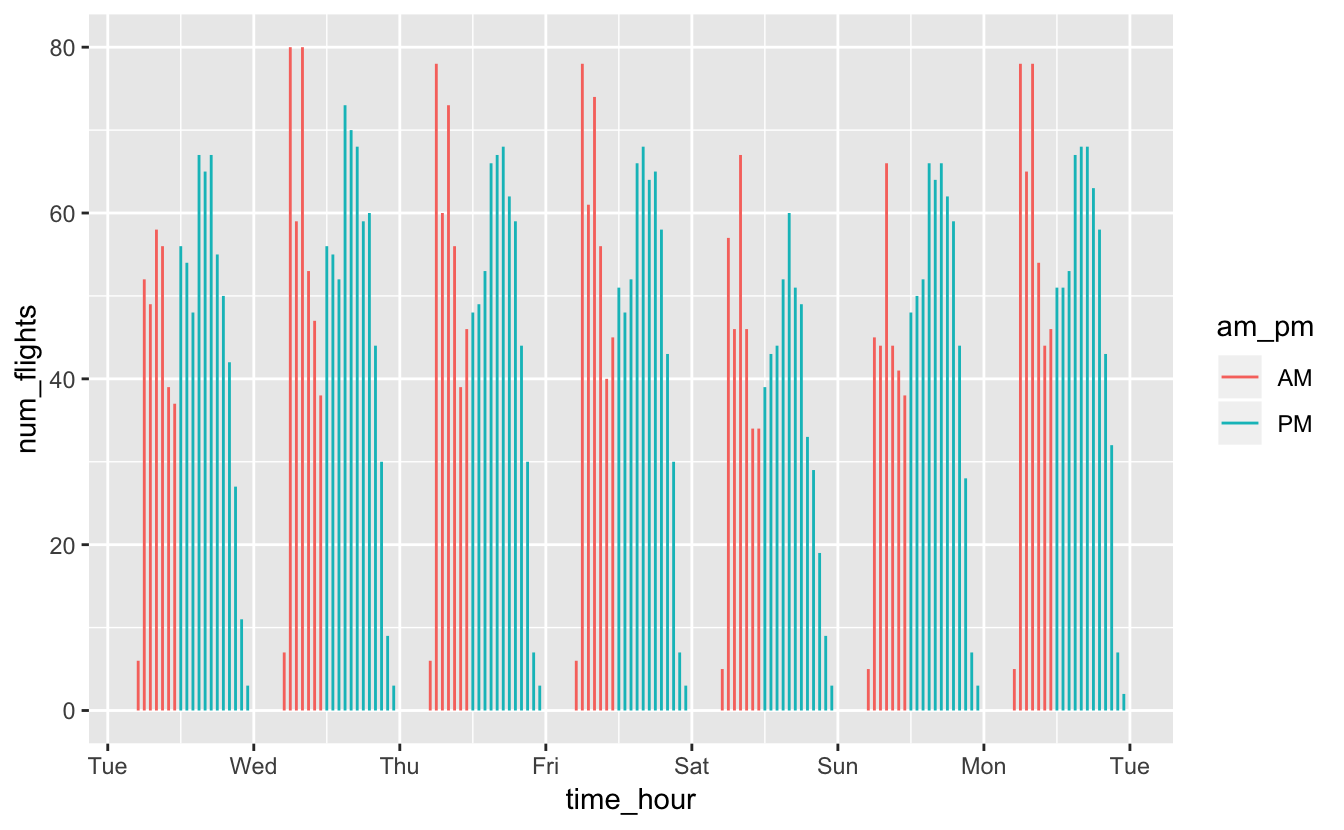In this case, there’s no long-term trend we’re interested in. Instead, we want to understand short-term fluctuations, and we care about individual values. In these situations, `geom_col()` and `geom_segment()` are good options.

## 6.4 Individual values

Sometimes, you’ll want to display time on the x-axis like a time series, but you won’t actually care about displaying any kind of trend.

The `famines` dataset from dcldata tracks major famines across time.

``````famines
#> # A tibble: 77 x 6
#>   location   iso_a3 region year_start year_end deaths_estimate
#>   <chr>      <chr>  <chr>       <dbl>    <dbl>           <dbl>
#> 1 Ireland    irl    Europe       1846     1852         1000000
#> 2 India      ind    Asia         1860     1861         2000000
#> 3 Cape Verde cpv    Africa       1863     1867           30000
#> 4 India      ind    Asia         1866     1867          961043
#> 5 Finland    fin    Europe       1868     1868          100000
#> 6 India      ind    Asia         1868     1870         1500000
#> # … with 71 more rows``````

There’s no obvious relationship between time and deaths due to famines.

``````famines %>%
ggplot(aes(year_start, deaths_estimate)) +
geom_point() +
scale_y_log10()``````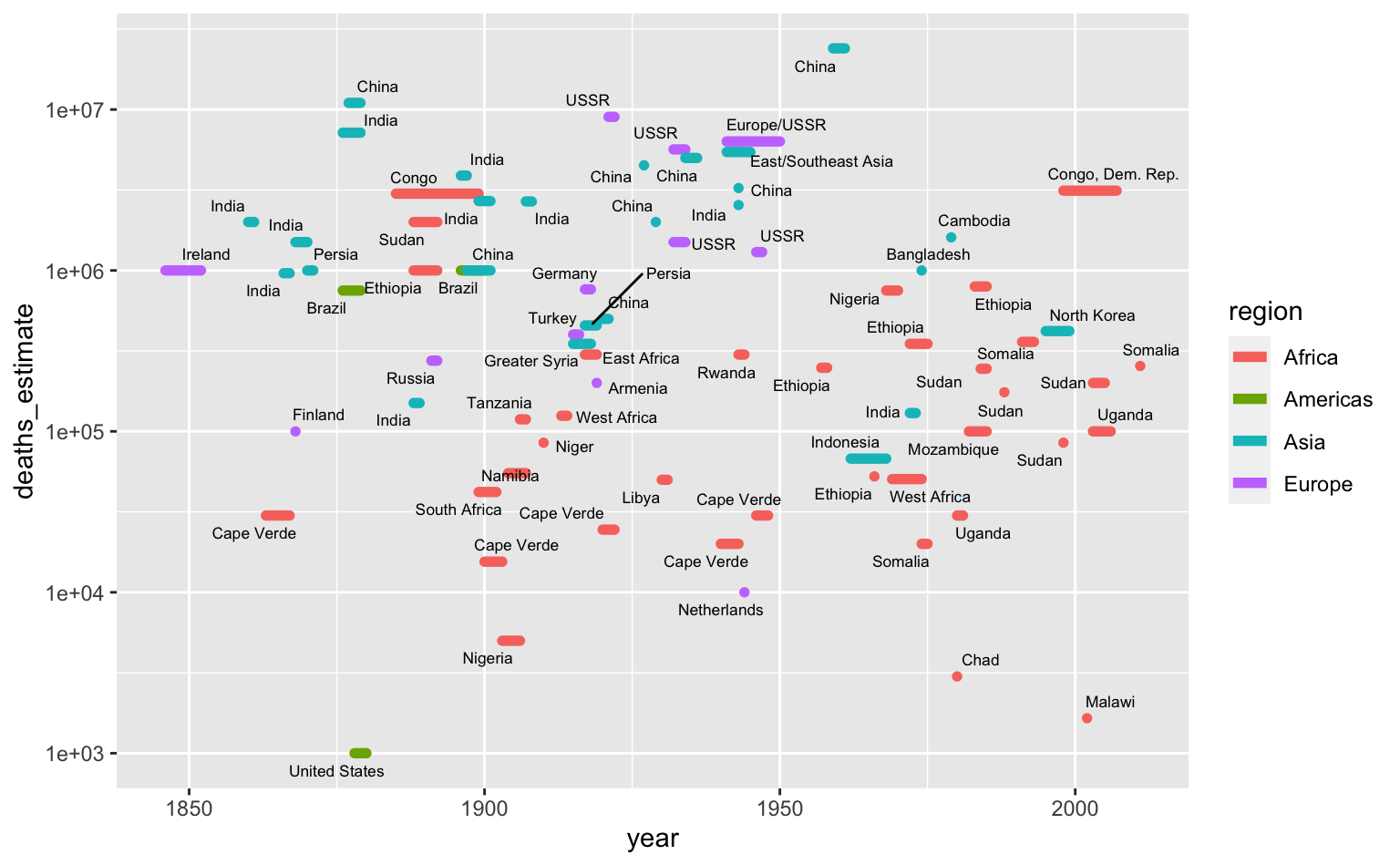Even though there’s no trend, this data is still interesting if you’re curious about individual famines.

The above plot only uses the `start` date, but we also have the length of the famines. We can treat the x-axis as representing year generally and encode the length of a line as the length of the famine.

``````famines %>%
arrange(desc(deaths_estimate)) %>%
ggplot(aes(year_start, deaths_estimate)) +
geom_segment(
aes(xend = year_end, yend = deaths_estimate, color = region),
size = 2,
lineend = "round"
) +
ggrepel::geom_text_repel(aes(label = location), size = 2.3) +
scale_y_log10() +
labs(x = "year")``````